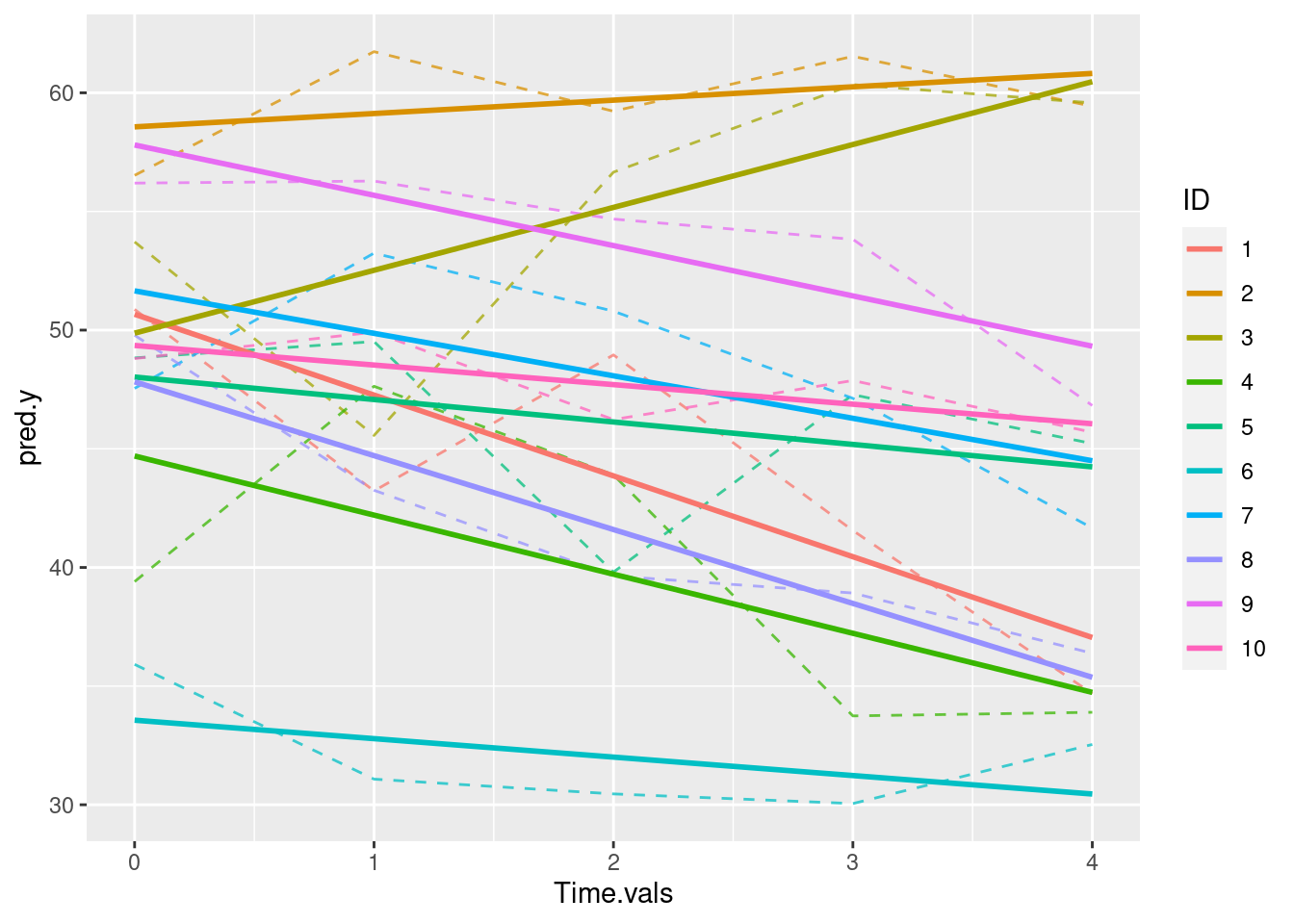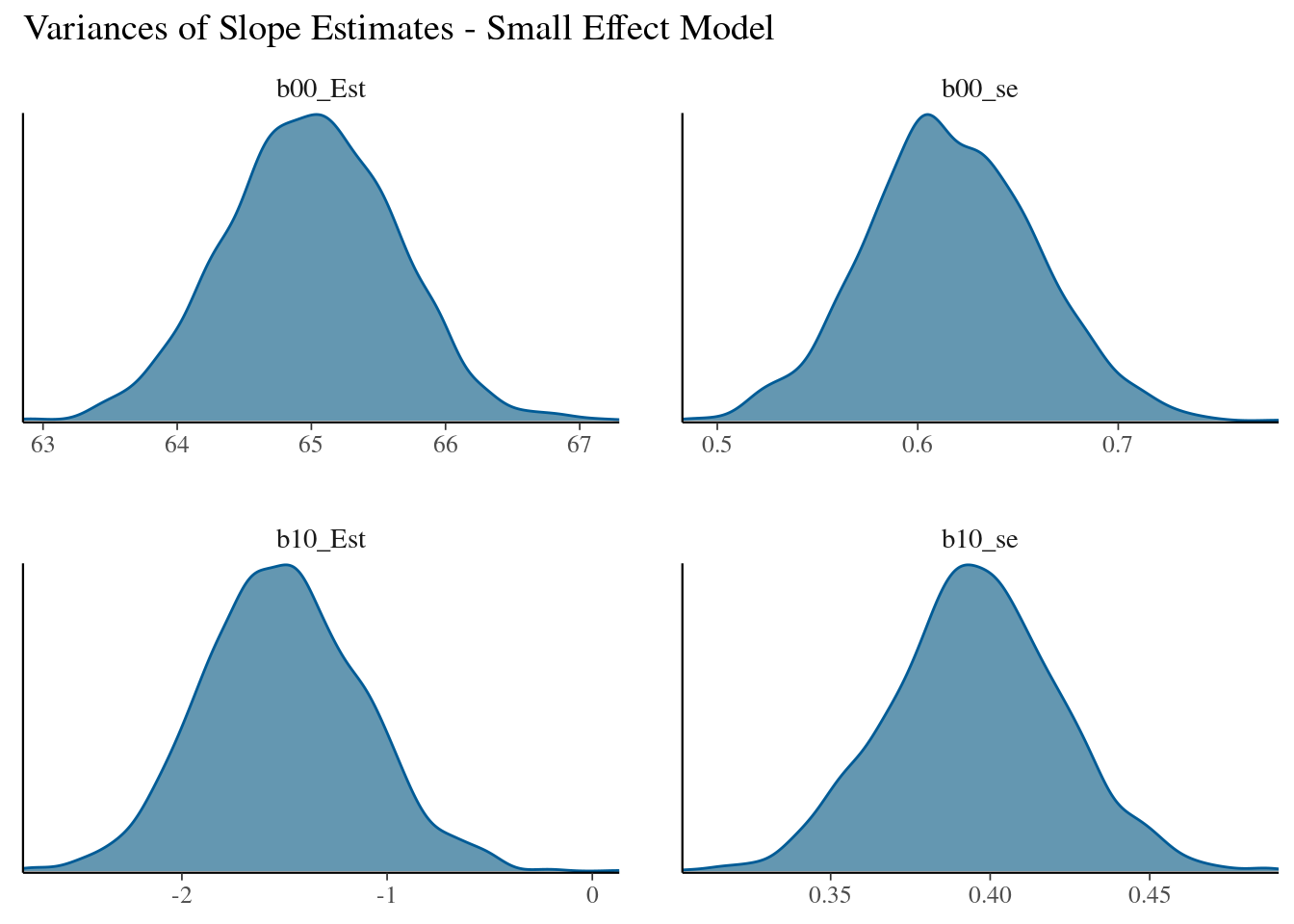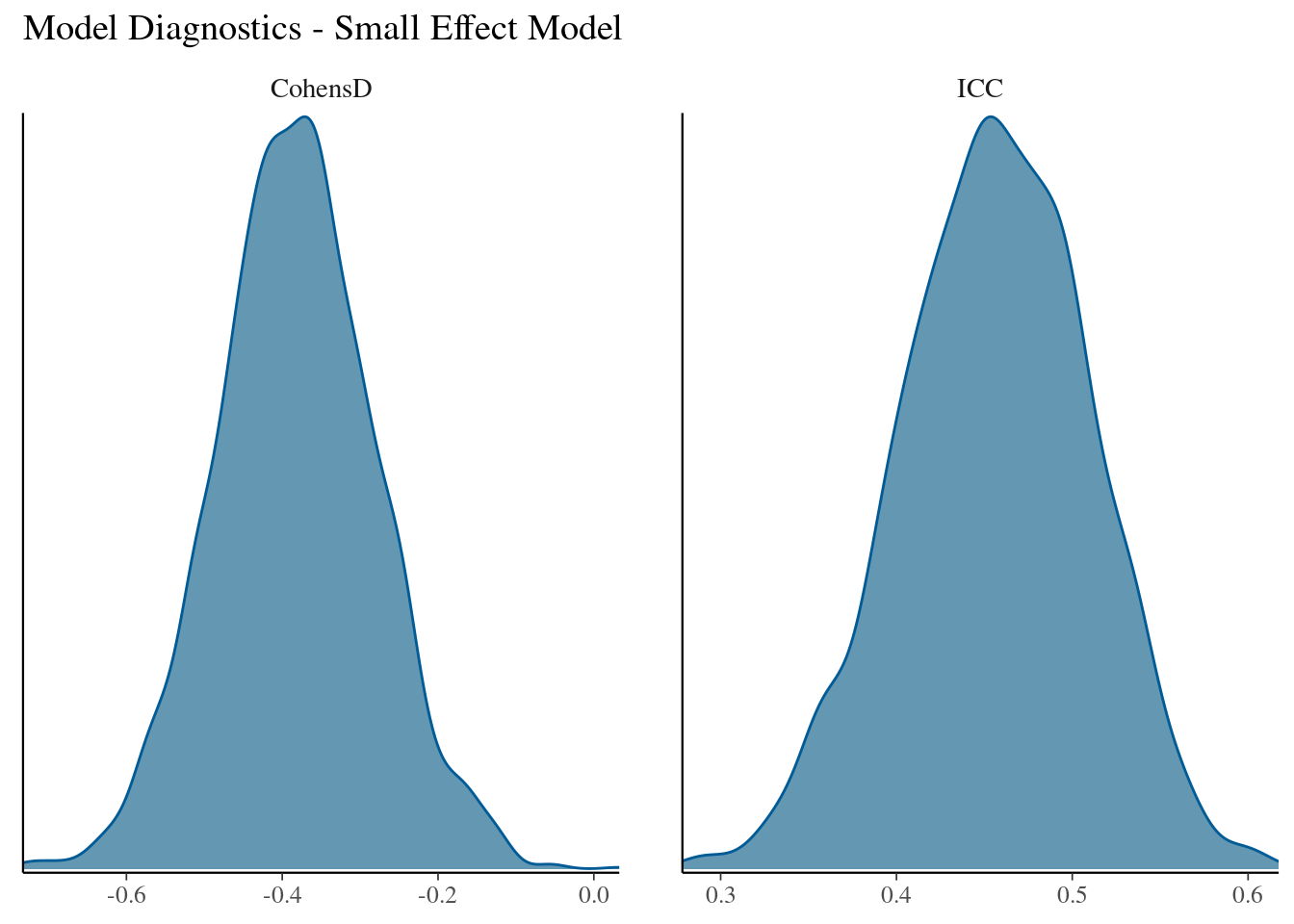# Power Analyses for an Unconditional Growth Model using {lmer}

By Matthew Barstead, Ph.D. | June 7, 2018

Recently, I was asked to knock together a quick power analysis for a linear growth model with approximately 120 subjects. Having already collected data (i.e., having a fixed sample size), the goal of the power analysis was to explore whether a sample of 120 subjects would be sufficient to detect significant linear change ($\alpha = .05$) for a secondary research question that was not part of the original proposal (we added collection of a set of variables partway through the data collection period).

We collected measures of these variables at three time points, approximately evenly spaced apart, and, for the purposes of these analyses, I decided to treat the data as if they were collected at precisely the same equally spaced interval for all participants. Though this is not technically true, it is sufficiently true for the purposes of these analyses. Modifying the code to take into account the actual difference in time between individual assessments is entirely possible and potentially important depending on your measures and design.

The Problem: In short, I need a reasonable population model for my data. Ideally, this model is grounded in both theory and empirical findings.

To create a reasonable population model, even for a relatively simple analysis (the present working example qualifies as such), I need to think through what I know about the treatment effects and the target population. For instance, we know that data in the present case were obatined from a selected sample of children, who were eligible to participate if their scores on a measure of early childhood anxiety risk exceeded the 85th percentile.

This knowledge provides useful information when considering population priors. For instance, I should expect the obtained sample to be drawn from a population elevated in anxiety and other, related, symptoms of emotional and/or behavioral maladaptation at time 1.

This is useful until I need to consider what I mean by “elevated,” at least insofar as how I will define it numerically in the model. To address this definitional issue, it is helpful to adopt a scale (sometimes a standardized scale is a particularly good option given the easy and obvious interpretation of scores).

For the present set of analyses, I am going to attempt to place my outcome measure on a pseudo-T-score (i.e., $\mu \approx 50, \sigma \approx 10$), that is approximately normally distributed in the population (note that I am referring to a clinical T-score not a t distribution). In a Bayesian sense, I am setting a prior regarding the distribution, and it is a convenient one in that it will allow me to define the sample using intercept and slope values that convey meaningful information about initial levels of the outcome variable and overall change.

So far I have only settled on a starting point (i.e., the intercept), but I have a number of parameters I need to consider specfying, and most importantly a number of parameters about which I am somewhat uncertain. To see what other parameters I need to consider, it is perhaps useful to review the simple linear growth model (specified below using Raudenbush & Bryk’s notation).

Level 1 Equation:
$Y_{ti} = \pi_{0i} + \pi_{1i}(Time) + e_{ti}$

The level 1 equation includes the coefficient $\pi_{0i}$ which represents the average predicted value for $Y$ when $Time=0$. For this reason researchers typically center their intercepts at a meaningful value. In the present analyses $Time$ is coded such that $T_1 = 0, T_2 = 1,$ and $T_3 = 2$. With this specification, the intercept ($\pi_{0i}$}) represents the predicted value for the outcome score ($Y$) prior to the start of the intervention (note that I am also including a random effect in this model specification - more on that below).

Average change over time is represented in the model by $\pi_{1i}$. Any one case, however, likely deviates to some degree from the average model of change. These deviations are sometimes referred to as random effects. In the context of a growth model, they represent the degree to an individual case’s slope (or intercept) deviates from the estimated average. Using Raudenbush & Bryk’s (2002) notation, these random effects are defined below, where the $\beta$’s represent the fixed effects and the $r$’s represent individual deviations from the estimated fixed effects.

Level 2 Equations:
$\pi_{0i} = \beta_{00} + r_{0i}$ $\pi_{1i} = \beta_{10} + r_{1i}$

As a quick toy example, let’s see what putting priors into practice actually means. Say I have 10 cases measured repeatedly over 5 equally spaced time points. Over the course of the entire data collection window, I expect that I will see an average decrease of -2.5 units in the outcome measure. Additionally, I expect that while most participants will exhibit negative change overall, it is possible that some cases will experience positive change - I want to make sure that my priors allow for this possibility in practice.

set.seed(5499)

N<-10
Time.vals<-rep(0:4, times=N)  #5 time points
ID<-rep(1:N, each=5)
pred.y<-vector()
Time<-0:4

#Hyperparamters - fixed effects for slope and intercept
b_00<-50
b_10<--0.5
for(n in 1:N){
#Level 1 error
e_ti<-rnorm(5, 0, 3)

#Level 2 sources of error
r_0i<-rnorm(1, 0, 5)
r_1i<-rnorm(1, 0, 1.5)

#Level 1 coefficients
pi_0i<-b_00+r_0i
pi_1i<-b_10+r_1i

#Outcome incoporating level 1 error
pred.y<-c(pred.y, pi_0i+pi_1i*Time+e_ti)
}

DF.temp<-data.frame(pred.y, ID, Time.vals)
DF.temp$ID<-as.factor(DF.temp$ID) #a little easier to work with a grouping factor here.
g1<-ggplot(data=DF.temp)+
geom_line(aes(x=Time.vals, y=pred.y, group = ID, color=ID), lty='dashed', alpha=.75)+
geom_smooth(aes(x=Time.vals, y=pred.y, group=ID, color=ID), method = 'lm', se=F)
g1Looking at the plot above, let’s see if I got what I wanted (note the dashed lines are the raw data for each case). While most cases did in fact decline on average, there are two cases for which scores on the outcome measure increased.

Whenever conducting a power analysis, to the degree possible, it is important to ensure that the assumptions 40+you are building into that analysis are meaningfully grounded in some way - typically tethering a rationale to theory, empirical reports, or both.

Though true, this framework encourages thinking at the level of the overall effect. We often turn to meta-analyses or other reviews that have attempt to describe or define the population-level effect. Less often do these sorts of reviews speak to individual variability from mean estimates.

The net result is that we sometimes struggle to define our expectations about variability. This is where plotting the data is so valuable in my mind. I can quickly see whether or not individual trajectories are departing wildly from what is reasonable. If so, I can tweak certain aspects of the model and re-inspect until I am satisfied with the results.

We always stress plotting your real data. Same goes for the made-up stuff too.

Once I have sufficiently tuned up my population model, the next trick is to simulate a sufficiently large number of data sets to estimate power for my proposed model of change. Briefly, I will note that I am not really addressing the possibility that there is a meaningful correlation between individual random effects. I’ll save that for a future post.

The Solution: Now that I have something of a framework created, I can apply it to my problem in a more direct fashion. I should state at the outset that this simulation is going to take some time. That is because I have chosen to evaluate significance using coverage of 0 by a 95% boostrapped confidence interval. I tend to prefer this over say a p-value generated by the lmer() function using the {lmerTest} library. This may be overkill, but it is the way I would typically assess whether coefficients in the model meaningfully differ from 0, so it is the approach I will be using to assess power as well.

My goal with the analysis presented below is to assess the power of the model to detect significant negative linear change with the expectation that the overall effect in the population is relatively small (I’ll be using Cohen’s $d$ as a guide for evaluating effect size.

#The libraries used
library(lme4)
library(merTools)
library(boot)
library(sjstats)
library(ggplot2)

#Specifying fixed effects for model
b_00<-65        #Fixed intercept: Average starting point on a pseudo-t-score scale)
b_10<--1.5      #Fixed slope: Change per unit of time
Time<-0:2       #Vector of equally-spaced intervals

#Setting up some empty vectors for the simulation to fill in
#------------------------------------------------------------------------------------
#Intercept vectors
b00_Est<-vector()
b00_boot_se<-vector()
b00_boot_LB<-vector()
b00_boot_UB<-vector()
b00_var<-vector()

#Slope vectors
b10_Est<-vector()
b10_boot_se<-vector()
b10_boot_LB<-vector()
b10_boot_UB<-vector()
b10_var<-vector()

#Capturing variability in Y at multiple levels and overall
ICC.vals<-vector()
sd.y<-vector()
CohensD<-vector()
#------------------------------------------------------------------------------------
#Select number of simulations & Sample size
n.sims<-2500  #number of total simulations to run - recommend > 5,000
N<-120          #Sample size

for(s in 1:n.sims){
#browser()
Time.vals<-rep(0:2, times=N)
IDs<-rep(1:N, each=3)
pred.y<-vector()
for(n in 1:N){
#Level 1 error
e_ti<-rnorm(3, 0, 5)

#Level 2 sources of error
r_0i<-rnorm(1, 0, 5)
r_1i<-rnorm(1, 0, 2.5)

#Level 1 coefficients
pi_0i<-b_00+r_0i
pi_1i<-b_10+r_1i

#Outcome incoporating level 1 error
pred.y<-c(pred.y, pi_0i+pi_1i*Time+e_ti)
}

DF<-data.frame(ID=IDs,
Time=Time.vals,
Y=pred.y)

fit.null<-lme4::lmer(Y~1+(1|ID), DF)
ICC.vals<-c(ICC.vals, as.numeric(sjstats::icc(fit.null)))
sd.y<-c(sd.y, sd(pred.y))
CohensD<-c(CohensD, effsize::cohen.d(c(DF$Y[DF$Time==2], DF$Y[DF$Time==0]), f=rep(c('T3', 'T1'), each=120))$estimate) fit.ucgm<-lme4::lmer(Y~1+Time + (1+Time|ID), data=DF) boot.ucgm<-bootMer(fit.ucgm, FUN=fixef, type = 'parametric', nsim=1000, parallel = 'multicore', ncpus=12) #obtaining CIs for intercept b00_Est<-c(b00_Est, mean(boot.ucgm$t[,1]))
b00_boot_se<-c(b00_boot_se, sd(boot.ucgm$t[,1])) b00_boot_LB<-c(b00_boot_LB, b00_Est[s]+qt(.975, N-1)*b00_boot_se[s]) b00_boot_UB<-c(b00_boot_UB, b00_Est[s]+qt(.025, N-1)*b00_boot_se[s]) #obtaining CIs for time slope b10_Est<-c(b10_Est, mean(boot.ucgm$t[,2]))
b10_boot_se<-c(b10_boot_se, sd(boot.ucgm$t[,2])) b10_boot_LB<-c(b10_boot_LB, b10_Est[s]+qt(.975, N-1)*b10_boot_se[s]) b10_boot_UB<-c(b10_boot_UB, b10_Est[s]+qt(.025, N-1)*b10_boot_se[s]) #Obtaining estimates of variability in slope and intercept b00_var<-c(b00_var, as.numeric(VarCorr(fit.ucgm)$ID[1,1]))
b10_var<-c(b10_var, as.numeric(VarCorr(fit.ucgm)$ID[2,2])) print(paste(s, 'out of', n.sims, 'simulations')) } Now that the simulation has finished, it is time to combine the output and plot. I cannot stress enough how important it is to plot things. Again, I am looking to see whether or not this model has returned reasonable estimates. (Pro tip: run your whole simulation code with a much smaller number of simulations to start - say 30 - inspect the results, then run the full simulation code if everything checks out.) dat_small<-data.frame(b00_Est=b00_Est, b00_se=b00_boot_se, b00_var=b00_var, b00_boot_LB = b10_boot_LB, b00_boot_UB = b10_boot_UB, b10_Est=b10_Est, b10_se=b10_boot_se, b10_var=b10_var, b10_boot_LB = b10_boot_LB, b10_boot_UB = b10_boot_UB, sd.y=sd.y, CohensD=CohensD, ICC=ICC.vals) #plotting distributions returned from the simulations #Selecting only certain columns related to fixed effects bayesplot::mcmc_dens(dat_small[,c(1:2,6:7)])+ ggtitle('Variances of Slope Estimates - Small Effect Model')What about some of the basic properties of the data? Was the average linear effect relatively small (which was the goal of this analysis after all)? What about the intra-class correlations returned by each data set; were they reasonable given the design and expectations based on similar data sets? bayesplot::mcmc_dens(dat_small[,12:13])+ ggtitle('Model Diagnostics - Small Effect Model')I would say yes to both. The maximum a posteriori estimate (MAPE) for standardized average change is: median(dat_small$CohensD)
##  -0.3826507
quantile(dat_small$CohensD, c(.025, .975)) ## 2.5% 97.5% ## -0.5780296 -0.1765590 which to me seems entirely reasonable to classify as a “small” effect power analysis, with the added bonus that you can clearly see this approach reflects uncertainty about the magnitude of change observed in any one sample. So how do I get power? It is simple. I am interested in the power to detect negative change at $\alpha = .05$, given the population model I created, in a sample of 120 subjects. Okay so not that simple. Since I saved the upper boundaries of the 95% CIs for my estimates I could add up all of the times the upper boundary for a given sample was less than 0 (an indication that a significant effect was detected), divide that total by the total number of simulations and voila, I’d have a calculation of power. Using a binary variable it is even easier… sig<-ifelse(dat_small$b10_boot_UB<0, 1, 0)
mean(sig)
##  1
max(dat_small\$b10_boot_UB)
##  -0.6303266

Note that the maximum upper boundary for model-based 95%-bootstrapped CIs for the fixed slope was negative - which is why I get a probability of 1 using the mean(sig) call. If interested I could use this information to start working my way down iteratively to a minimum effect size detectable at a rate of 80%, given the population model and a sample size of 120.

Or… Instead of doing all of that trial and error work, I could create a program to do it, one that has its code written more efficiently, and one that does a little more parallelizing. All for a future post.